# Disperse systems

Filed under Basic Science

rate 1 star rate 2 star rate 3 star rate 4 star rate 5 star# Disperse systems

David Attwood

Chapter contents

## Introduction

A disperse system consists essentially of one component, the disperse phase, dispersed as particles or droplets throughout another component, the continuous phase. By definition, those dispersions in which the size of the dispersed particles is within the range 10−9 m (1 nm) to about 10−6 m (1 µm) are termed colloidal. However, the upper size limit is often extended to include emulsions and suspensions which are very polydisperse systems in which the droplet size frequently exceeds 1 µm, but which show many of the properties of colloidal systems. Some examples of colloidal systems of pharmaceutical interest are shown in Table 5.1. Many natural systems such as suspensions of microorganisms, blood and isolated cells in culture are also colloidal dispersions.

Table 5.1

Types of disperse systems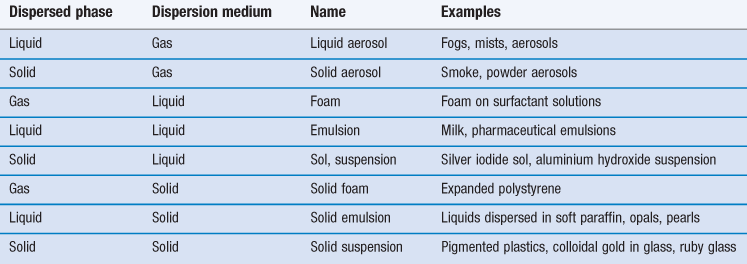This chapter will examine the properties of both coarse dispersions, such as emulsions, suspensions and aerosols, and also fine dispersions, such as micellar systems, which fall within the defined size range of true colloidal dispersions.

Colloids can be broadly classified as those that are lyophobic (solvent-hating) and those that are lyophilic (solvent-liking). The terms hydrophobic and hydrophilic are used when the solvent is water. Surfactant molecules tend to associate in water into aggregates called micelles and these constitute hydrophilic colloidal dispersions. Proteins and gums also form lyophilic colloidal systems because of a similar affinity between the dispersed particles and the continuous phase. On the other hand, dispersions of oil droplets in water or water droplets in oil are examples of lyophobic dispersions.

It is because of the subdivision of matter in colloidal systems that they have special properties. A common feature of these systems is a large surface-to-volume ratio of the dispersed particles. As a consequence, there is a tendency for the particles to associate in order to reduce their surface area. Emulsion droplets, for example, eventually coalesce to form a macrophase, so attaining a minimum surface area and hence an equilibrium state. This chapter will examine how the stability of colloidal dispersions can be understood by a consideration of the forces acting between the dispersed particles. Approaches to the formulation of emulsions, suspensions and aerosols will be described and the instability of these coarse dispersions will be discussed using a theory of colloid stability. The association of surface-active agents into micelles and the applications of these colloidal dispersions in the solubilization of poorly water-soluble drugs will also be considered.

## Colloids

### Preparation of colloidal systems

#### Lyophilic colloids

The affinity of lyophilic colloids for the dispersion medium leads to the spontaneous formation of colloidal dispersions. For example, acacia, tragacanth, methylcellulose and certain other cellulose derivatives readily disperse in water. This simple method of dispersion is a general one for the formation of lyophilic colloids.

#### Lyophobic colloids

The preparative methods for lyophobic colloids may be divided into those methods that involve the breakdown of larger particles into particles of colloidal dimensions (dispersion methods) and those in which the colloidal particles are formed by aggregation of smaller particles such as molecules (condensation methods).

###### Dispersion methods.

The breakdown of coarse material may be carried out by the use of a colloid mill or ultrasonics.

###### Colloid mills.

These mills cause the dispersion of coarse material by shearing in a narrow gap between a static cone (the stator) and a rapidly rotating cone (the rotor).

###### Ultrasonic treatment.

The passage of ultrasonic waves through a dispersion medium produces alternating regions of cavitation and compression in the medium. The cavities collapse with great force and cause the breakdown of coarse particles dispersed in the liquid.

With both these methods the particles will tend to reunite unless a stabilizing agent such as a surface-active agent is added.

###### Condensation methods.

These involve the rapid production of supersaturated solutions of the colloidal material under conditions in which it is deposited in the dispersion medium as colloidal particles and not as a precipitate. The supersaturation is often obtained by means of a chemical reaction that results in the formation of the colloidal material. For example, colloidal silver iodide may be obtained by reacting together dilute solutions of silver nitrate and potassium iodide; colloidal sulphur is produced from sodium thiosulfate and hydrochloric acid solutions; and ferric chloride boiled with excess of water produces colloidal hydrated ferric oxide.

A change of solvent may also cause the production of colloidal particles by condensation methods. If a saturated solution of sulphur in acetone is poured slowly into hot water, the acetone vaporizes, leaving a colloidal dispersion of sulphur. A similar dispersion may be obtained when a solution of a resin, such as benzoin in alcohol, is poured into water.

### Purification of colloidal systems

#### Dialysis

Colloidal particles are not retained by conventional filter papers but are too large to diffuse through the pores of membranes such as those made from regenerated cellulose products, e.g. collodion (cellulose nitrate evaporated from a solution in alcohol and ether) and cellophane. The smaller molecules in solution are able to pass through these membranes. Use is made of this difference in diffusibility to separate micromolecular impurities from colloidal dispersions. The process is known as dialysis. The process of dialysis may be hastened by stirring so as to maintain a high concentration gradient of diffusible molecules across the membrane and by renewing the outer liquid from time to time.

###### Ultrafiltration.

By applying pressure (or suction), the solvent and small particles may be forced across a membrane whilst the larger colloidal particles are retained. The process is referred to as ultrafiltration. It is possible to prepare membrane filters with known pore size and use of these allows the particle size of a colloid to be determined. However, particle size and pore size cannot be properly correlated because the membrane permeability is affected by factors such as electrical repulsion, when both the membrane and particle carry the same charge, and particle adsorption which can lead to blocking of the pores.

###### Electrodialysis.

An electric potential may be used to increase the rate of movement of ionic impurities through a dialysing membrane and so provide a more rapid means of purification. The concentration of charged colloidal particles at one side and at the base of the membrane is termed electrodecantation.

### Properties of colloids

#### Size and shape of colloidal particles

###### Size distribution.

Within the size range of colloidal dimensions specified above, there is often a wide distribution of sizes of the dispersed colloidal particles. The molecular weight or particle size is therefore an average value, the magnitude of which is dependent on the experimental technique used in its measurement. When determined by the measurement of colligative properties such as osmotic pressure, a number average value, Mn, is obtained which, in a mixture containing n1, n2, n3, … moles of particle of mass M1, M2, M3, … respectively, is defined by: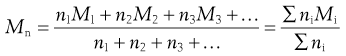(5.1)

In the light-scattering method for the measurement of particle size, larger particles produce greater scattering and the weight rather than the number of particles is important, giving a weight-average value, Mw, defined by: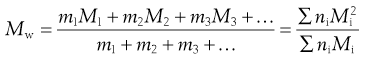(5.2)

In Equation 5.2, m1, m2, and m3 … are the masses of each species, and mi is obtained by multiplying the mass of each species by the number of particles of that species; that is, mi = niMi. A consequence is that Mw > Mn, and only when the system is monodisperse will the two averages be identical. The ratio Mw/Mn expresses the degree of polydispersity of the system.

###### Shape.

Many colloidal systems, including emulsions, liquid aerosols and most dilute micellar solutions, contain spherical particles. Small deviations from sphericity are often treated using ellipsoidal models. Ellipsoids of revolution are characterized by their axial ratio, which is the ratio of the half-axis a to the radius of revolution b (see Fig. 5.1). Where this ratio is greater than unity, the ellipsoid is said to be a prolate ellipsoid (rugby ball shaped), and when less than unity an oblate ellipsoid (discus-shaped).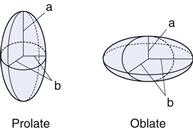High molecular weight polymers and naturally occurring macromolecules often form random coils in aqueous solution. Clay suspensions are examples of systems containing plate-like particles.

#### Kinetic properties

In this section several properties of colloidal systems, which relate to the motion of particles with respect to the dispersion medium, will be considered. Thermal motion manifests itself in the form of Brownian motion, diffusion and osmosis. Gravity (or a centrifugal field) leads to sedimentation. Viscous flow is the result of an externally applied force. Measurement of these properties enables molecular weights or particle size to be determined.

###### Brownian motion.

Colloidal particles are subject to random collisions with the molecules of the dispersion medium with the result that each particle pursues an irregular and complicated zigzag path. If the particles (up to about 2 µm diameter) are observed under a microscope or the light scattered by colloidal particles is viewed using an ultramicroscope, an erratic motion is seen. This movement is referred to as Brownian motion after Robert Brown who first reported his observation of this phenomenon with pollen grains suspended in water.

###### Diffusion.

As a result of Brownian motion, colloidal particles spontaneously diffuse from a region of higher concentration to one of lower concentration. The rate of diffusion is expressed by Fick’s First Law. One form of this relationship is shown in Equation 5.3.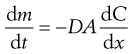(5.3)

where dm is the mass of substance diffusing in time dt across an area A under the influence of a concentration gradient dC/dx (the minus sign denotes that diffusion takes place in the direction of decreasing concentration). D is the diffusion coefficient and has the dimensions of area per unit time. The diffusion coefficient of a dispersed material is related to the frictional coefficient, f, of the particles by Einstein’s Law of Diffusion:(5.4)

where kB is the Boltzmann constant and T temperature.

Therefore, as the frictional coefficient is given by the Stokes equation: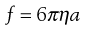(5.5)

where η is the viscosity of the medium and a the radius of the particle (assuming sphericity), then: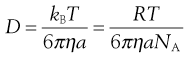(5.6)

NA is the Avogadro constant, R is the universal gas constant and kB = R/NA. The diffusion coefficient may be obtained by an experiment measuring the change in concentration, via refractive index gradients, when the solvent is carefully layered over the solution to form a sharp boundary and diffusion is allowed to proceed. A more commonly used method is that of dynamic light scattering which is based on the frequency shift of laser light as it is scattered by a moving particle, the so-called Doppler shift. The diffusion coefficient can be used to obtain the molecular weight of an approximately spherical particle, such as egg albumin and haemoglobin, by using Equation 5.5 in the form: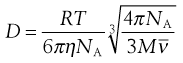(5.7)

where M is the molecular weight and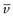is the partial specific volume of the colloidal material.

###### Sedimentation.

Consider a spherical particle of radius a and density σ falling in a liquid of density ρ and viscosity η. The velocity v of sedimentation is given by Stokes’ Law: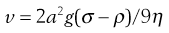(5.8)

where g is acceleration due to gravity.

If the particles are only subjected to the force of gravity then, due to Brownian motion, the lower size limit of particles obeying Equation 5.8 is about 0.5 µm. A stronger force than gravity is therefore needed for colloidal particles to sediment and use is made of a high-speed centrifuge, usually termed an ultracentrifuge, which can produce a force of about 106 g. In a centrifuge, g is replaced by ω2x, where ω is the angular velocity and x the distance of the particle from the centre of rotation.

The ultracentrifuge is used in two distinct ways in investigating colloidal material. In the sedimentation velocity method, a high centrifugal field is applied, up to about 4 × 105 g, and the movement of the particles, monitored by changes in concentration, is measured at specified time intervals. In the sedimentation equilibrium method, the colloidal material is subjected to a much lower centrifugal field until sedimentation and diffusion tendencies balance one another, and an equilibrium distribution of particles throughout the sample is attained.

###### Sedimentation velocity.

The velocity dx/dt of a particle in a unit centrifugal force can be expressed in terms of the Svedberg coefficient s: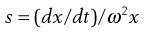(5.9)

Under the influence of the centrifugal force, particles pass from position x1 at time t1 to position x2 at time t2. The differences in concentration with time can be measured using changes in refractive index and the application of the schlieren optical arrangement, whereby photographs can be taken showing these concentrations as peaks. The expression giving molecular weight M from this method is: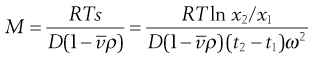(5.10)

whereis the partial specific volume of the particle.

###### Sedimentation equilibrium.

Equilibrium is established when sedimentation and diffusional forces balance.

Combination of sedimentation and diffusion equations is made in the analysis giving: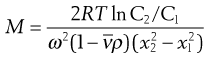(5.11)

where C1 and C2 are the sedimentation equilibrium concentrations at distances x1 and x2 from the axis of rotation. A disadvantage of the sedimentation equilibrium method is the length of time required to attain equilibrium, often as long as several days. A modification of the method in which measurements are made in the early stages of the approach to equilibrium significantly reduces the overall measurement time.

###### Osmotic pressure.

The determination of molecular weights of dissolved substances from colligative properties such as the depression of freezing point or the elevation of boiling point is a standard procedure. However, of the available methods, only osmotic pressure has a practical value in the study of colloidal particles because of the magnitude of the changes in the properties. For example, the depression of freezing point of a 1% w/v solution of a macromolecule of molecular weight 70 000 Da is only 0.0026 K, far too small to be measured with sufficient accuracy by conventional methods and also very sensitive to the presence of low molecular weight impurities. In contrast, the osmotic pressure of this solution at 20 °C would be 350 N m−2 or about 35 mm of water. Not only does the osmotic pressure provide an effect that is measurable, but also the effect of any low molecular weight material, which can pass through the membrane, is virtually eliminated.

However, the usefulness of osmotic pressure measurement is limited to a molecular weight range of about 104−106 Da; below 104 Da the membrane may be permeable to the molecules under consideration and above 106 Da the osmotic pressure will be too small to permit accurate measurement.

If a solution and solvent are separated by a semi-permeable membrane, the tendency to equalize chemical potentials (and hence concentrations) on either side of the membrane results in a net diffusion of solvent across the membrane. The pressure necessary to balance this osmotic flow is termed the osmotic pressure.

For a colloidal solution the osmotic pressure, Π, can be described by: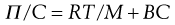(5.12)

where C is the concentration of the solution, M the molecular weight of the solute and B a constant depending on the degree of interaction between the solvent and solute molecules.

Thus a plot of Π/C versus C is linear with the value of the intercept at C → 0 giving RT/M enabling the molecular weight of the colloid to be calculated. The molecular weight obtained from osmotic pressure measurements is a number-average value.

A potential source of error in the determination of molecular weight from osmotic pressure measurements arises from the Donnan membrane effect. The diffusion of small ions through a membrane will be affected by the presence of a charged macromolecule that is unable to penetrate the membrane because of its size. At equilibrium, the distribution of the diffusible ions is unequal, being greater on the side of the membrane containing the non-diffusible ions. Consequently, unless precautions are taken to correct for this effect or eliminate it, the results of osmotic pressure measurements on charged colloidal particles such as proteins will be invalid.

###### Viscosity.

Viscosity is an expression of the resistance to flow of a system under an applied stress. An equation of flow applicable to colloidal dispersions of spherical particles was developed by Einstein: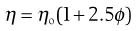(5.13)

where ηo is the viscosity of the dispersion medium and η the viscosity of the dispersion when the volume fraction of colloidal particles present is ϕ.

A number of viscosity coefficients may be defined with respect to Equation 5.13. These include relative viscosity: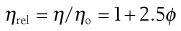(5.14)

and specific viscosity: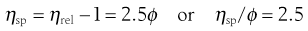(5.15)

Since volume fraction is directly related to concentration, Equation 5.15 may be written as: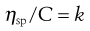(5.16)

where C is the concentration expressed as grams of colloidal particles per 100 mL of total dispersion and k is a constant. If η is determined for a number of concentrations of macromolecular material in solution and ηsp/C is plotted versus C then the intercept obtained on extrapolation of the linear plot to infinite dilution is known as the intrinsic viscosity [η].

This constant may be used to calculate the molecular weight of the macromolecular material by making use of the Mark–Houwink equation: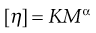(5.17)

where K and α are constants characteristic of the particular polymer-solvent system. These constants are obtained initially by determining [η] for a polymer fraction whose molecular weight has been determined by another method such as sedimentation, osmotic pressure or light scattering. The molecular weight of the unknown polymer fraction may then be calculated. This method is suitable for use with polymers, such as dextrans used as blood plasma substitutes.

#### Optical properties

###### Light scattering.

When a beam of light is passed through a colloidal sol (dispersion of very fine particles), some of the light may be absorbed (when light of certain wavelengths is selectively absorbed, a colour is produced), some is scattered and the remainder is transmitted undisturbed through the sample. Due to the light scattered, the sol appears turbid; this is known as the Tyndall effect. The turbidity of a sol is given by the expression: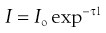(5.18)

where Io is the intensity of the incident beam, I that of the transmitted light beam, l the length of the sample and τ the turbidity.

Light-scattering measurements are of great value for estimating particle size, shape and interactions, particularly of dissolved macromolecular materials, as the turbidity depends on the size (molecular weight) of the colloidal material involved. Measurements are simple in principle but experimentally difficult because of the need to keep the sample free from dust, the particles of which would scatter light strongly and introduce large errors.

As most colloids show very low turbidities, instead of measuring the transmitted light (which may differ only marginally from the incident beam), it is more convenient and accurate to measure the scattered light, at an angle (usually 90°) relative to the incident beam. The turbidity can then be calculated from the intensity of the scattered light, provided the dimensions of the particle are small compared to the wavelength of the incident light, by the expression: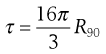(5.19)

R90 is known as the Rayleigh ratio after Lord Rayleigh who laid the foundations of the light-scattering theory. The light-scattering theory was modified for use in the determination of the molecular weight of colloidal particles by Debye who derived the following relationship between turbidity and molecular weight:(5.20)

C is the concentration of the solute and B an interaction constant allowing for non-ideality. H is an optical constant for a particular system depending on the refractive index change with concentration and the wavelength of light used. A plot of HC/τ against concentration results in a straight line of slope 2B. The intercept on the HC/τ axis is 1/M, allowing the molecular weight to be calculated. The molecular weight derived by the light-scattering technique is a weight-average value.

Light-scattering measurements are particularly suitable for finding the size of the micelles of surface-active agents and for the study of proteins and natural and synthetic polymers.

For spherical particles, the upper limit of the Debye equation is a particle diameter of approximately one-twentieth of the wavelength λ of the incident light; that is, about 20–25 nm. The light-scattering theory becomes more complex when one or more dimensions exceed λ/20 because the particles can no longer be considered as point sources of scattered light. By measuring the light scattering from such particles as a function of both the scattering angle θ and the concentration C, and extrapolating the data to zero angle and zero concentration, it is possible to obtain information on not only the molecular weight but also the particle shape.

Because the intensity of the scattered light is inversely proportional to the fourth power of the wavelength of the light used, blue light (λ = 450 nm) is scattered much more than red light (λ = 650 nm). With incident white light, a scattering material will therefore tend to be blue when viewed at right angles to the incident beam, which is why the sky appears to be blue, the scattering arising from dust particles in the atmosphere.

###### Ultramicroscopy.

Colloidal particles are too small to be seen with an optical microscope. Light scattering is employed in the ultramicroscope first developed by Zsigmondy, in which a cell containing the colloid is viewed against a dark background at right angles to an intense beam of incident light. The particles, which exhibit Brownian motion, appear as spots of light against the dark background. The ultramicroscope is used in the technique of microelectrophoresis for measuring particle charge.

###### Electron microscopy.

The electron microscope, capable of giving actual pictures of the particles, is used to observe the size, shape and structure of colloidal particles. The success of the electron microscope is due to its high resolving power, defined in terms of d, the smallest distance by which two objects are separated yet remain distinguishable. The smaller the wavelength of the radiation used, the smaller is d and the greater the resolving power. An optical microscope, using visible light as its radiation source, gives a d of about 0.2 µm. The radiation source of the electron microscope is a beam of high-energy electrons having wavelengths in the region of 0.01 nm; d is thus about 0.5 nm. The electron beams are focused using electromagnets and the whole system is under a high vacuum of about 10−3–10−5 Pa to give the electrons a free path. With wavelengths of the order indicated, the image cannot be viewed directly, so the image is displayed on a monitor or computer screen.

A major disadvantage of the electron microscope for viewing colloidal particles is that normally only dried samples can be examined. Consequently, it usually gives no information on solvation or configuration in solution and, moreover, the particles may be affected by sample preparation. A recent development which overcomes these problems is environmental scanning electron microscopy (ESEM) which allows the observation of material in the wet state.

#### Electrical properties

###### Electrical properties of interfaces.

Most surfaces acquire a surface electric charge when brought into contact with an aqueous medium, the principal charging mechanisms being as follows.

###### Ion dissolution.

Ionic substances can acquire a surface charge by virtue of unequal dissolution of the oppositely charged ions of which they are composed. For example, the particles of silver iodide in a solution with excess [I] will carry a negative charge, but the charge will be positive if excess [Ag+] is present. Since the concentrations of Ag+ and I determine the electric potential at the particle surface, they are termed potential-determining ions. In a similar way, H+ and OH are potential-determining ions for metal oxides and hydroxides of, for example, magnesium and aluminium hydroxides.

###### Ionization.

Here the charge is controlled by the ionization of surface groupings; examples include the model system of polystyrene latex which frequently has carboxylic acid groupings at the surface which ionize to give negatively charged particles. In a similar way, acidic drugs such as ibuprofen and nalidixic acid also acquire a negative charge.

Amino acids and proteins acquire their charge mainly through the ionization of carboxyl and amino groups to give —COO and NH3+ ions. The ionization of these groups and so the net molecular charge depends on the pH of the system. At a pH below the pKa of the COO group the protein will be positively charged because of the protonation of this group, —COO → COOH, and the ionization of the amino group, —NH2 → —NH3+, which has a much higher pKa. At higher pH, where the amino group is no longer ionized, the net charge on the molecule is negative because of the ionization of the carboxyl group. At a certain definite pH, specific for each individual protein, the total number of positive charges will equal the total number of negative charges and the net charge will be zero. This pH is termed the isoelectric point of the protein and the protein exists as its zwitterion. This may be represented as follows: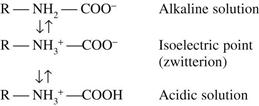A protein is least soluble (the colloidal sol is least stable) at its isoelectric point and is readily desolvated by very water-soluble salts such as ammonium sulfate. Thus insulin may be precipitated from aqueous alcohol at pH 5.2.

A net surface charge can be acquired by the unequal adsorption of oppositely charged ions. Surfaces in water are more often negatively charged than positively charged, because cations are generally more hydrated than anions. Consequently, the former have the greater tendency to reside in the bulk aqueous medium whereas the smaller, less hydrated and more polarizing anions have the greater tendency to reside at the particle surface. Surface-active agents are strongly adsorbed and have a pronounced influence on the surface charge, imparting either a positive or negative charge depending on their ionic character.

###### The electrical double layer.

Consider a solid charged surface in contact with an aqueous solution containing positive and negative ions. The surface charge influences the distribution of ions in the aqueous medium; ions of opposite charge to that of the surface, termed counter-ions, are attracted towards the surface, ions of like charge, termed co-ions, are repelled away from the surface. However, the distribution of the ions will also be affected by thermal agitation which will tend to redisperse the ions in solution. The result is the formation of an electrical double layer made up of the charged surface and a neutralizing excess of counter-ions over co-ions (the system must be electrically neutral) distributed in a diffuse manner in the aqueous medium.

The theory of the electric double layer deals with this distribution of ions and hence with the magnitude of the electric potentials which occur in the locality of the charged surface. For a fuller explanation of what is a rather complicated mathematical approach, the reader is referred to a textbook of colloid science (e.g. Shaw 1992). A somewhat simplified picture of what pertains from the theories of Gouy, Chapman and Stern follows.

The double layer is divided into two parts (see Fig. 5.2a): the inner, which may include adsorbed ions, and the diffuse part where ions are distributed as influenced by electrical forces and random thermal motion. The two parts of the double layer are separated by a plane, the Stern plane, at about a hydrated ion radius from the surface; thus counter-ions may be held at the surface by electrostatic attraction and the centre of these hydrated ions forms the Stern plane.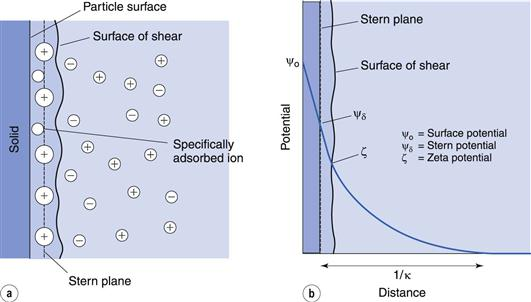The potential changes linearly from ψo (the surface potential) to ψδ, (the Stern potential) in the Stern layer and decays exponentially from ψδ to zero in the diffuse double layer (Fig. 5.2b). A plane of shear is also indicated in Figure 5.2. In addition to ions in the Stern layer, a certain amount of solvent will be bound to the ions and the charged surface. This solvating layer is held to the surface, and the edge of the layer, termed the surface or plane of shear, represents the boundary of relative movement between the solid (and attached material) and the liquid. The potential at the plane of shear is termed the zeta, ζ, or electrokinetic, potential and its magnitude may be measured using microelectrophoresis or any other of the electrokinetic phenomena. The thickness of the solvating layer is ill-defined and the zeta potential therefore represents a potential at an unknown distance from the particle surface; its value, however, is usually taken as being slightly less than that of the Stern potential.

In the discussion above, it was stated that the Stern plane existed at a hydrated ion radius from the particle surface; the hydrated ions are electrostatically attracted to the particle surface. It is possible for ions/molecules to be more strongly adsorbed at the surface, termed specific adsorption, than by simple electrostatic attraction. In fact, the specifically adsorbed ion/molecule may be uncharged as is the case with non-ionic surface-active agents. Surface-active ions specifically adsorb by the hydrophobic effect and can have a significant effect on the Stern potential, causing ψo and ψδ to have opposite signs, as in Figure 5.3a, or for ψδ to have the same sign as ψo but be greater in magnitude, as in Figure 5.3b.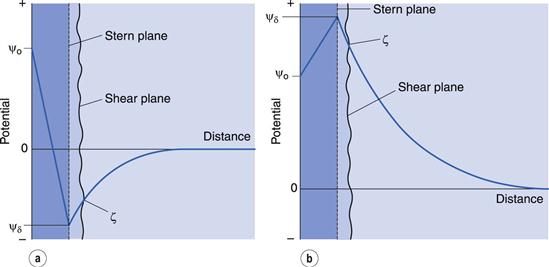Figure 5.2b shows an exponential decay of the potential to zero with distance from the Stern plane. The distance over which this occurs is 1/κ, referred to as the Debye–Hückel length parameter or the thickness of the electrical double layer. The parameter κ is dependent on the electrolyte concentration of the aqueous media. Increasing the electrolyte concentration increases the value of κ and consequently decreases the value of 1/κ; that is, it compresses the double layer. As ψδ stays constant this means that the zeta potential will be lowered.

As indicated earlier, the effect of specifically adsorbed ions may be to lower the Stern potential and hence the zeta potential without compressing the double layer. Thus the zeta potential may be reduced by additives to the aqueous system in either (or both) of two different ways.

###### Electrokinetic phenomena.

This is the general description applied to the phenomena that arise when attempts are made to shear off the mobile part of the electrical double layer from a charged surface. There are four such phenomena: namely, electrophoresis, sedimentation potential, streaming potential and electroosmosis. All of these electrokinetic phenomena may be used to measure the zeta potential but electrophoresis is the easiest to use and has the greatest pharmaceutical application.

###### Electrophoresis.

The movement of a charged particle (plus attached ions) relative to a stationary liquid under the influence of an applied electric field is termed electrophoresis. When the movement of the particles is observed with a microscope, or the movement of light spots scattered by particles too small to be observed with the microscope is observed using an ultramicroscope, this constitutes microelectrophoresis.

A microscope equipped with an eyepiece graticule is used and the speed of movement of the particle under the influence of a known electric field is measured. This is the electrophoretic velocity, v, and the electrophoretic mobility, u, is given by: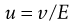(5.21)

where v is measured in m s−1, and E, the applied field strength, in V m−1, so that u has the dimensions of m2 s−1 V−1. Typically, a stable lyophobic colloidal particle may have an electrophoretic mobility of 4 × 10−8 m2 s−1 V−1. The equation used for converting the electrophoretic mobility, u, into the zeta potential depends on the value of κa (κ is the Debye–Hückel reciprocal length parameter described previously and a the particle radius). For values of κa > 100 (as is the case for particles of radius 1 µm dispersed in 10−3 mol dm−3 sodium chloride solution) the Smoluchowski equation can be used: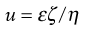(5.22)

where ε is the permittivity and η the viscosity of the liquid used. For particles in water at 25 °C, ζ = 12.85 × 10−5 u volts and, for the mobility given above, a zeta potential of 0.0514 volts or 51.4 millivolts is obtained. For values of κa < 100, a more complex relationship which is a function of κa and the zeta potential is used.

The technique of microelectrophoresis finds application in the measurement of zeta potentials, of model systems (like polystyrene latex dispersions) to test colloid stability theory, of coarse dispersions (like suspensions and emulsions) to assess their stability, and in identification of charge groups and other surface characteristics of water-insoluble drugs and cells such as blood and bacteria.

Other electrokinetic phenomena The other electrokinetic phenomena are as follows. Sedimentation potential